### Home > A2C > Chapter 10 > Lesson 10.2.3 > Problem10-99

10-99.
1. Simplify each expression below. Think about how you could use rules of exponents, the distributive property, and the multiplicative property of one to find shortcuts. Homework Help ✎

1. 3−2 · 34

2. 3−4 · 34

3. (3−1+3−3) · 34

4.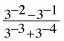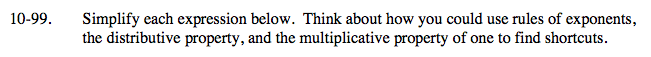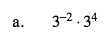3−2 · 34 = 3(4 − 2) = 9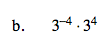See part (a).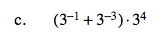34 · 3−1 + 34 · 3−3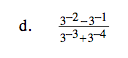$\frac{3^{4}}{3^{4}}\cdot \frac{3^{-2}-3^{-1}}{3^{-3}+3^{-4}}$

$-\frac{9}{2}$